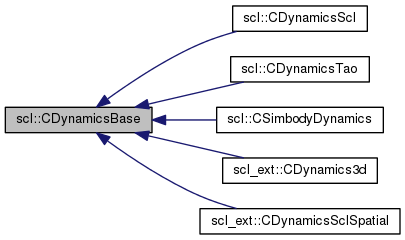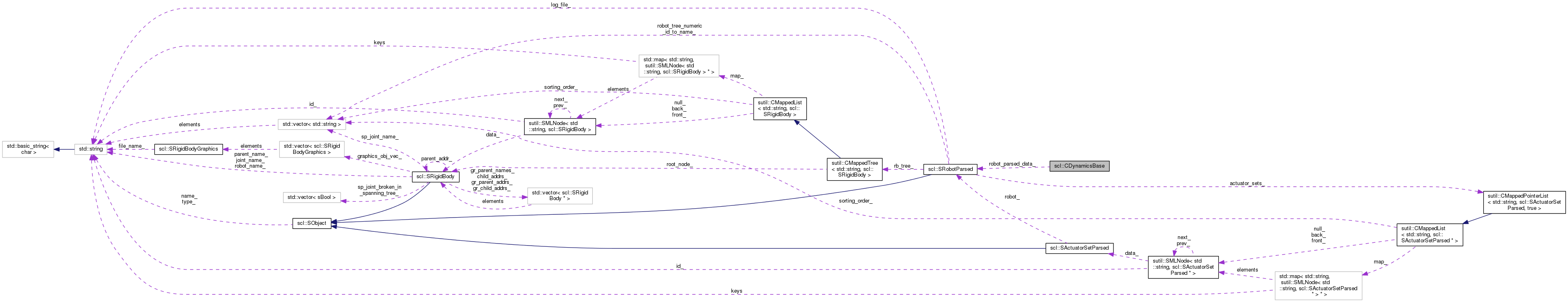SCL  1.0 Standard Control Library : Control, dynamics, physics, and simulation
scl::CDynamicsBase Class Referenceabstract

#include <CDynamicsBase.hpp>

Inheritance diagram for scl::CDynamicsBase:[legend]
Collaboration diagram for scl::CDynamicsBase:[legend]

Public Member Functions

virtual sBool computeGCModel (const SRobotSensors *arg_sensor_data, SGcModel *arg_gc_model)=0

virtual sBool computeTransformsForAllLinks (sutil::CMappedTree< std::string, SRigidBodyDyn > &arg_tree, const Eigen::VectorXd &arg_q)

virtual sBool computeTransform (SRigidBodyDyn &arg_link, const Eigen::VectorXd &arg_q)

virtual sBool computeTransformToAncestor (Eigen::Affine3d &arg_T, SRigidBodyDyn &arg_link, const SRigidBodyDyn *arg_ancestor, const Eigen::VectorXd &arg_q)

virtual sBool computeJacobianWithTransforms (Eigen::MatrixXd &arg_J, SRigidBodyDyn &arg_link, const Eigen::VectorXd &arg_q, const Eigen::Vector3d &arg_pos_local)

virtual sBool computeJacobian (Eigen::MatrixXd &arg_J, const SRigidBodyDyn &arg_link, const Eigen::VectorXd &arg_q, const Eigen::Vector3d &arg_pos_local) const

virtual sBool computeJacobian (Eigen::MatrixXd &arg_J, const SRigidBodyDyn &arg_link, const SRigidBodyDyn *arg_ancestor, const Eigen::VectorXd &arg_q, const Eigen::Vector3d &arg_pos_local)

virtual sBool computeJacobianTrans (Eigen::MatrixXd &arg_J, const SRigidBodyDyn &arg_link, const SRigidBodyDyn *arg_ancestor, const Eigen::VectorXd &arg_q, const Eigen::Vector3d &arg_pos_local)

virtual sBool computeJacobianRot (Eigen::MatrixXd &arg_J, const SRigidBodyDyn &arg_link, const SRigidBodyDyn *arg_ancestor, const Eigen::VectorXd &arg_q, const Eigen::Vector3d &arg_pos_local)

virtual sBool computeExternalContacts (sutil::CMappedList< std::string, SForce > &arg_contacts)

virtual sBool integrate (SRobotIO &arg_inputs, const sFloat arg_time_interval)

virtual sFloat computeEnergyKinetic (sutil::CMappedTree< std::string, SRigidBodyDyn > &arg_tree, const Eigen::VectorXd &arg_q, const Eigen::VectorXd &arg_dq)

virtual sFloat computeEnergyPotential (sutil::CMappedTree< std::string, SRigidBodyDyn > &arg_tree, const Eigen::VectorXd &arg_q)

CDynamicsBase ()

virtual ~CDynamicsBase ()

virtual sBool init (const SRobotParsed &arg_robot_data)=0

virtual sBool hasBeenInit ()

Protected Attributes

sBool has_been_init_

const SRobotParsedrobot_parsed_data_

Detailed Description

A base class for different dynamics implementations.

Using any controller requires supporting this api with a dynamics engine implementation.

All dynamics implementations must try to be stateless. Ie. After one initial configuration that specifies the dynamic model's parameters, they must not store any more data. Pass in the model configuration as an argument and return the new state and/or dynamics matrices.

Constructor & Destructor Documentation

 scl::CDynamicsBase::CDynamicsBase ( )
inline

Default constructor sets the initialization state to false

 virtual scl::CDynamicsBase::~CDynamicsBase ( )
inlinevirtual

Default destructor does nothing

Member Function Documentation

 virtual sFloat scl::CDynamicsBase::computeEnergyKinetic ( sutil::CMappedTree< std::string, SRigidBodyDyn > & arg_tree, const Eigen::VectorXd & arg_q, const Eigen::VectorXd & arg_dq )
inlinevirtual

Gets the robot's kinetic energy

Parameters
 arg_tree The tree for which the transformation matrices are to be updated arg_q The current generalized coordinates. arg_dq The current generalized velocities.

Reimplemented in scl::CDynamicsScl, scl_ext::CDynamics3d, and scl_ext::CDynamicsSclSpatial.

 virtual sFloat scl::CDynamicsBase::computeEnergyPotential ( sutil::CMappedTree< std::string, SRigidBodyDyn > & arg_tree, const Eigen::VectorXd & arg_q )
inlinevirtual

Gets the robot's potential energy

Parameters
 arg_tree The tree for which the transformation matrices are to be updated arg_q The current generalized coordinates.

Reimplemented in scl::CDynamicsScl, scl_ext::CDynamicsSclSpatial, and scl_ext::CDynamics3d.

 virtual sBool scl::CDynamicsBase::computeExternalContacts ( sutil::CMappedList< std::string, SForce > & arg_contacts )
inlinevirtual

Calculates the collision forces for the robot to which this dynamics object is assigned.

Uses std::string based force lookup. The dynamics implementation should support this (maintain a map or something).

NOTE : The dynamics engine may delete forces from the passed mapped list at will. Do not store pointers to them at random places in your code.

As of now, this is optional for dynamics engines

Parameters
 arg_contacts This is where the simulator will store the contact forces. It may use the std::string to identify when to remove or re-add forces.

Reimplemented in scl_ext::CDynamics3d.

 virtual sBool scl::CDynamicsBase::computeGCModel ( const SRobotSensors * arg_sensor_data, SGcModel * arg_gc_model )
pure virtual

Updates the generalized coordinate model matrices (Everything in SGcModel).

This is the most efficient method to access the standard matrices. Computing transformations and Jacobians individually is typically wasteful.

Parameters
 arg_sensor_data Current robot state. q, dq, ddq, sensed generalized forces and perceived external forces. arg_gc_model Individual link Jacobians, and composite intertial, centrifugal/coriolis gravity estimates.
 virtual sBool scl::CDynamicsBase::computeJacobian ( Eigen::MatrixXd & arg_J, const SRigidBodyDyn & arg_link, const Eigen::VectorXd & arg_q, const Eigen::Vector3d & arg_pos_local ) const
inlinevirtual

Calculates the Jacobian for the robot to which this dynamics object is assigned. dx_global_origin = Jx . dq The Jacobian is specified by a link and an offset (in task space dimensions)from that link. ** CONST VERSION : NOTE : The regular version should call this. **

Parameters
 arg_J The Jacobain will be saved here. arg_link The link at which the Jacobian is to be calculated arg_q The current generalized coordinates. arg_pos_local The offset from the link's frame (in link coordinates).

Reimplemented in scl::CDynamicsScl.

 virtual sBool scl::CDynamicsBase::computeJacobian ( Eigen::MatrixXd & arg_J, const SRigidBodyDyn & arg_link, const SRigidBodyDyn * arg_ancestor, const Eigen::VectorXd & arg_q, const Eigen::Vector3d & arg_pos_local )
inlinevirtual

Calculates the Jacobian for the robot to which this dynamics object is assigned. dx_ancestor_coords = Jx . dq The Jacobian is specified by a link and an offset (in task space dimensions)from that link.

Parameters
 arg_J The Jacobain will be saved here. arg_link The link at which the Jacobian is to be calculated arg_ancestor The link up to which the Jacobian is to be calculated Pass NULL to compute the Jacobian up to the global root. arg_q The current generalized coordinates. arg_pos_local The offset from the link's frame (in link coordinates).
 virtual sBool scl::CDynamicsBase::computeJacobianRot ( Eigen::MatrixXd & arg_J, const SRigidBodyDyn & arg_link, const SRigidBodyDyn * arg_ancestor, const Eigen::VectorXd & arg_q, const Eigen::Vector3d & arg_pos_local )
inlinevirtual

Calculates the Jacobian for the robot to which this dynamics object is assigned. ONLY for rotation. Ie. size(Jx) = (3,ndof) dx_ancestor_coords = Jx . dq

Parameters
 arg_J The Jacobain will be saved here. arg_link The link at which the Jacobian is to be calculated arg_ancestor The link up to which the Jacobian is to be calculated Pass NULL to compute the Jacobian up to the global root. arg_q The current generalized coordinates. arg_pos_local The offset from the link's frame (in link coordinates).
 virtual sBool scl::CDynamicsBase::computeJacobianTrans ( Eigen::MatrixXd & arg_J, const SRigidBodyDyn & arg_link, const SRigidBodyDyn * arg_ancestor, const Eigen::VectorXd & arg_q, const Eigen::Vector3d & arg_pos_local )
inlinevirtual

Calculates the Jacobian for the robot to which this dynamics object is assigned. ONLY for translation. Ie. size(Jx) = (3,ndof) dx_ancestor_coords = Jx . dq

Parameters
 arg_J The Jacobain will be saved here. arg_link The link at which the Jacobian is to be calculated arg_ancestor The link up to which the Jacobian is to be calculated Pass NULL to compute the Jacobian up to the global root. arg_q The current generalized coordinates. arg_pos_local The offset from the link's frame (in link coordinates).
 virtual sBool scl::CDynamicsBase::computeJacobianWithTransforms ( Eigen::MatrixXd & arg_J, SRigidBodyDyn & arg_link, const Eigen::VectorXd & arg_q, const Eigen::Vector3d & arg_pos_local )
inlinevirtual

Calculates the Jacobian for the robot to which this dynamics object is assigned. dx_global_origin = Jx . dq The Jacobian is specified by a link and an offset (in task space dimensions)from that link.

Parameters
 arg_J The Jacobain will be saved here. arg_link The link at which the Jacobian is to be calculated. Transforms are updated in this link and ancestors in the tree. arg_q The current generalized coordinates. arg_pos_local The offset from the link's frame (in link coordinates).

Reimplemented in scl::CDynamicsScl.

 virtual sBool scl::CDynamicsBase::computeTransform ( SRigidBodyDyn & arg_link, const Eigen::VectorXd & arg_q )
inlinevirtual

Calculates the Transformation Matrix for the robot to which this dynamics object is assigned. x_parent_link_coords = arg_link.T_lnk_ * x_link_coords Note that the matrix is stored in the passed link data struct.

Parameters
 arg_link The link at which the transformation matrix is to be calculated arg_q The current generalized coordinates.

Reimplemented in scl::CDynamicsScl.

 virtual sBool scl::CDynamicsBase::computeTransformsForAllLinks ( sutil::CMappedTree< std::string, SRigidBodyDyn > & arg_tree, const Eigen::VectorXd & arg_q )
inlinevirtual

Parameters
 arg_tree The tree for which the transformation matrices are to be updated arg_q The current generalized coordinates.

Reimplemented in scl::CDynamicsScl.

 virtual sBool scl::CDynamicsBase::computeTransformToAncestor ( Eigen::Affine3d & arg_T, SRigidBodyDyn & arg_link, const SRigidBodyDyn * arg_ancestor, const Eigen::VectorXd & arg_q )
inlinevirtual

Calculates the Transformation Matrix for the robot to which this dynamics object is assigned. x_ancestor_link_coords = arg_link.T_lnk_ * x_link_coords

Note that the local transformation matrices are updated in all the link data structs from arg_link to arg_ancestor.

Also note that there are two origins, robot origin, which actually has a node allocated for it. And the global scl origin, for which you should pass in a NULL as ancestor.

Parameters
 arg_T The transformation matrix in which to store the answer arg_link The link at which the transformation matrix is to be calculated arg_ancestor The link up to which the transformation matrix is to be calculated Pass NULL to compute the transform up to the global root. arg_q The current generalized coordinates.

Reimplemented in scl::CDynamicsScl.

 virtual sBool scl::CDynamicsBase::hasBeenInit ( )
inlinevirtual

Initialization state

 virtual sBool scl::CDynamicsBase::init ( const SRobotParsed & arg_robot_data )
pure virtual

Initializes the dynamics to be computed for a specific robot.

Returns, true : Succeeds in creating a dynamics object

false : If it can't find the robot or if the information is inconsistent with what the implementation requires, it returns false

NOTE TODO : Should strive for a stateless dynamics implementation...

 virtual sBool scl::CDynamicsBase::integrate ( SRobotIO & arg_inputs, const sFloat arg_time_interval )
inlinevirtual

Integrates the robot's state. Uses the given applied forces, torques, positions and velocities and its internal dynamic model to compute new positions and velocities. Operates on the SRobotIO data structure.

Parameters
 arg_inputs The existing generalized coordinates, velocities and accelerations + The generalized forces + task (euclidean) forces and the list of contact points and links. arg_time_interval The time across which the system should integrate the dynamics. Could take fixed steps or dynamic ones in between. Up to the integrator implementation.

Reimplemented in scl::CSimbodyDynamics, scl::CDynamicsTao, scl_ext::CDynamics3d, and scl_ext::CDynamicsSclSpatial.

Member Data Documentation

 sBool scl::CDynamicsBase::has_been_init_
protected

True if the dynamics object has been initialized for a given robot

 const SRobotParsed* scl::CDynamicsBase::robot_parsed_data_
protected

A read-only pointer to access parsed data

The documentation for this class was generated from the following file: